matlab与数字图像处理_数字图像处理的数字图像处理的matlab实现 - CSDN

•matlab 图像处理
• ## 数字图像处理matlab版

千次阅读 多人点赞 2018-12-08 13:25:41
转载自：... 1.图像反转 MATLAB程序实现如下： I=imread('xian.bmp'); J=double(I); J=-J+(256-1); %图像反转线性变换 H=uint8(J); subplot(1,2,1),imshow(I); subpl...
转载自：http://blog.csdn.net/u012116229/article/details/44775277
1.图像反转
MATLAB程序实现如下：
I=imread('xian.bmp');
J=double(I);
J=-J+(256-1);                 %图像反转线性变换
H=uint8(J);
subplot(1,2,1),imshow(I);
subplot(1,2,2),imshow(H);

2.灰度线性变换
MATLAB程序实现如下：
I=imread('xian.bmp');
subplot(2,2,1),imshow(I);
title('原始图像');
axis([50,250,50,200]);
axis on;                  %显示坐标系
I1=rgb2gray(I);
subplot(2,2,2),imshow(I1);
title('灰度图像');
axis([50,250,50,200]);
axis on;                  %显示坐标系
subplot(2,2,3),imshow(J);
title('线性变换图像[0.1 0.5]');
axis([50,250,50,200]);
grid on;                  %显示网格线
axis on;                  %显示坐标系
subplot(2,2,4),imshow(K);
title('线性变换图像[0.3 0.7]');
axis([50,250,50,200]);
grid on;                  %显示网格线
axis on;                  %显示坐标系

3.非线性变换
MATLAB程序实现如下：
I=imread('xian.bmp');
I1=rgb2gray(I);
subplot(1,2,1),imshow(I1);
title('灰度图像');
axis([50,250,50,200]);
grid on;                  %显示网格线
axis on;                  %显示坐标系
J=double(I1);
J=40*(log(J+1));
H=uint8(J);
subplot(1,2,2),imshow(H);
title('对数变换图像');
axis([50,250,50,200]);
grid on;                  %显示网格线
axis on;                  %显示坐标系

4.直方图均衡化
MATLAB程序实现如下：
I=imread('xian.bmp');
I=rgb2gray(I);
figure;
subplot(2,2,1);
imshow(I);
subplot(2,2,2);
imhist(I);
I1=histeq(I);
figure;
subplot(2,2,1);
imshow(I1);
subplot(2,2,2);
imhist(I1);

5.线性平滑滤波器
用MATLAB实现领域平均法抑制噪声程序：
I=imread('xian.bmp');
subplot(231)
imshow(I)
title('原始图像')
I=rgb2gray(I);
I1=imnoise(I,'salt & pepper',0.02);
subplot(232)
imshow(I1)
title('添加椒盐噪声的图像')
k1=filter2(fspecial('average',3),I1)/255;          %进行3*3模板平滑滤波
k2=filter2(fspecial('average',5),I1)/255;          %进行5*5模板平滑滤波
k3=filter2(fspecial('average',7),I1)/255;          %进行7*7模板平滑滤波
k4=filter2(fspecial('average',9),I1)/255;          %进行9*9模板平滑滤波
subplot(233),imshow(k1);title('3*3模板平滑滤波');
subplot(234),imshow(k2);title('5*5模板平滑滤波');
subplot(235),imshow(k3);title('7*7模板平滑滤波');
subplot(236),imshow(k4);title('9*9模板平滑滤波');

6.中值滤波器
用MATLAB实现中值滤波程序如下：
I=imread('xian.bmp');
I=rgb2gray(I);
J=imnoise(I,'salt&pepper',0.02);
subplot(231),imshow(I);title('原图像');
subplot(232),imshow(J);title('添加椒盐噪声图像');
k1=medfilt2(J);            %进行3*3模板中值滤波
k2=medfilt2(J,[5,5]);      %进行5*5模板中值滤波
k3=medfilt2(J,[7,7]);      %进行7*7模板中值滤波
k4=medfilt2(J,[9,9]);      %进行9*9模板中值滤波
subplot(233),imshow(k1);title('3*3模板中值滤波');
subplot(234),imshow(k2);title('5*5模板中值滤波');
subplot(235),imshow(k3);title('7*7模板中值滤波');
subplot(236),imshow(k4);title('9*9模板中值滤波');

7.用Sobel算子和拉普拉斯对图像锐化：
I=imread('xian.bmp');
subplot(2,2,1),imshow(I);
title('原始图像');
axis([50,250,50,200]);
grid on;                  %显示网格线
axis on;                  %显示坐标系
I1=im2bw(I);
subplot(2,2,2),imshow(I1);
title('二值图像');
axis([50,250,50,200]);
grid on;                  %显示网格线
axis on;                  %显示坐标系
H=fspecial('sobel');     %选择sobel算子
J=filter2(H,I1);            %卷积运算
subplot(2,2,3),imshow(J);
title('sobel算子锐化图像');
axis([50,250,50,200]);
grid on;                  %显示网格线
axis on;                  %显示坐标系
h=[0 1 0,1 -4 1,0 1 0];   %拉普拉斯算子
J1=conv2(I1,h,'same');            %卷积运算
subplot(2,2,4),imshow(J1);
title('拉普拉斯算子锐化图像');
axis([50,250,50,200]);
grid on;                  %显示网格线
axis on;                  %显示坐标系

8.梯度算子检测边缘
用MATLAB实现如下：
I=imread('xian.bmp');
subplot(2,3,1);
imshow(I);
title('原始图像');
axis([50,250,50,200]);
grid on;                  %显示网格线
axis on;                  %显示坐标系
I1=im2bw(I);
subplot(2,3,2);
imshow(I1);
title('二值图像');
axis([50,250,50,200]);
grid on;                  %显示网格线
axis on;                  %显示坐标系
I2=edge(I1,'roberts');
figure;
subplot(2,3,3);
imshow(I2);
title('roberts算子分割结果');
axis([50,250,50,200]);
grid on;                  %显示网格线
axis on;                  %显示坐标系
I3=edge(I1,'sobel');
subplot(2,3,4);
imshow(I3);
title('sobel算子分割结果');
axis([50,250,50,200]);
grid on;                  %显示网格线
axis on;                  %显示坐标系
I4=edge(I1,'Prewitt');
subplot(2,3,5);
imshow(I4);
title('Prewitt算子分割结果');
axis([50,250,50,200]);
grid on;                  %显示网格线
axis on;                  %显示坐标系

9.LOG算子检测边缘
用MATLAB程序实现如下：
I=imread('xian.bmp');
subplot(2,2,1);
imshow(I);
title('原始图像');
I1=rgb2gray(I);
subplot(2,2,2);
imshow(I1);
title('灰度图像');
I2=edge(I1,'log');
subplot(2,2,3);
imshow(I2);
title('log算子分割结果');

10.Canny算子检测边缘
用MATLAB程序实现如下：
I=imread('xian.bmp');
subplot(2,2,1);
imshow(I);
title('原始图像')
I1=rgb2gray(I);
subplot(2,2,2);
imshow(I1);
title('灰度图像');
I2=edge(I1,'canny');
subplot(2,2,3);
imshow(I2);
title('canny算子分割结果');

11.边界跟踪（bwtraceboundary函数）
clc
clear all
figure
imshow(I);
title('原始图像');
I1=rgb2gray(I);                %将彩色图像转化灰度图像
threshold=graythresh(I1);        %计算将灰度图像转化为二值图像所需的门限
BW=im2bw(I1, threshold);       %将灰度图像转化为二值图像
figure
imshow(BW);
title('二值图像');
dim=size(BW);
col=round(dim(2)/2)-90;         %计算起始点列坐标
row=find(BW(:,col),1);          %计算起始点行坐标
connectivity=8;
num_points=180;
contour=bwtraceboundary(BW,[row,col],'N',connectivity,num_points);
%提取边界
figure
imshow(I1);
hold on;
plot(contour(:,2),contour(:,1), 'g','LineWidth' ,2);
title('边界跟踪图像');

12.Hough变换
I= imread('xian.bmp');
rotI=rgb2gray(I);
subplot(2,2,1);
imshow(rotI);
title('灰度图像');
axis([50,250,50,200]);
grid on;
axis on;
BW=edge(rotI,'prewitt');
subplot(2,2,2);
imshow(BW);
title('prewitt算子边缘检测后图像');
axis([50,250,50,200]);
grid on;
axis on;
[H,T,R]=hough(BW);
subplot(2,2,3);
imshow(H,[],'XData',T,'YData',R,'InitialMagnification','fit');
title('霍夫变换图');
xlabel('\theta'),ylabel('\rho');
axis on , axis normal, hold on;
P=houghpeaks(H,5,'threshold',ceil(0.3*max(H(:))));
x=T(P(:,2));y=R(P(:,1));
plot(x,y,'s','color','white');
lines=houghlines(BW,T,R,P,'FillGap',5,'MinLength',7);
subplot(2,2,4);,imshow(rotI);
title('霍夫变换图像检测');
axis([50,250,50,200]);
grid on;
axis on;
hold on;
max_len=0;
for k=1:length(lines)
xy=[lines(k).point1;lines(k).point2];
plot(xy(:,1),xy(:,2),'LineWidth',2,'Color','green');
plot(xy(1,1),xy(1,2),'x','LineWidth',2,'Color','yellow');
plot(xy(2,1),xy(2,2),'x','LineWidth',2,'Color','red');
len=norm(lines(k).point1-lines(k).point2);
if(len>max_len)
max_len=len;
xy_long=xy;
end
end
plot(xy_long(:,1),xy_long(:,2),'LineWidth',2,'Color','cyan');

13.直方图阈值法
用MATLAB实现直方图阈值法：
I=imread('xian.bmp');
I1=rgb2gray(I);
figure;
subplot(2,2,1);
imshow(I1);
title('灰度图像')
axis([50,250,50,200]);
grid on;                  %显示网格线
axis on;                  %显示坐标系
[m,n]=size(I1);                            %测量图像尺寸参数
GP=zeros(1,256);                           %预创建存放灰度出现概率的向量
for k=0:255
GP(k+1)=length(find(I1==k))/(m*n);    %计算每级灰度出现的概率，将其存入GP中相应位置
end
subplot(2,2,2),bar(0:255,GP,'g')                   %绘制直方图
title('灰度直方图')
xlabel('灰度值')
ylabel('出现概率')
I2=im2bw(I,150/255);
subplot(2,2,3),imshow(I2);
title('阈值150的分割图像')
axis([50,250,50,200]);
grid on;                  %显示网格线
axis on;                  %显示坐标系
I3=im2bw(I,200/255);   %
subplot(2,2,4),imshow(I3);
title('阈值200的分割图像')
axis([50,250,50,200]);
grid on;                  %显示网格线
axis on;                  %显示坐标系

14.自动阈值法：Otsu法
用MATLAB实现Otsu算法：
  clc
clear all
subplot(1,2,1),imshow(I);
title('原始图像')
axis([50,250,50,200]);
grid on;                  %显示网格线
axis on;                  %显示坐标系
level=graythresh(I);     %确定灰度阈值
BW=im2bw(I,level);
subplot(1,2,2),imshow(BW);
title('Otsu法阈值分割图像')
axis([50,250,50,200]);
grid on;                  %显示网格线
axis on;                  %显示坐标系

15.膨胀操作
I=imread('xian.bmp');          %载入图像
I1=rgb2gray(I);
subplot(1,2,1);
imshow(I1);
title('灰度图像')
axis([50,250,50,200]);
grid on;                  %显示网格线
axis on;                  %显示坐标系
se=strel('disk',1);          %生成圆形结构元素
I2=imdilate(I1,se);             %用生成的结构元素对图像进行膨胀
subplot(1,2,2);
imshow(I2);
title('膨胀后图像');
axis([50,250,50,200]);
grid on;                  %显示网格线
axis on;                  %显示坐标系

16.腐蚀操作
MATLAB实现腐蚀操作
I=imread('xian.bmp');          %载入图像
I1=rgb2gray(I);
subplot(1,2,1);
imshow(I1);
title('灰度图像')
axis([50,250,50,200]);
grid on;                  %显示网格线
axis on;                  %显示坐标系
se=strel('disk',1);       %生成圆形结构元素
I2=imerode(I1,se);        %用生成的结构元素对图像进行腐蚀
subplot(1,2,2);
imshow(I2);
title('腐蚀后图像');
axis([50,250,50,200]);
grid on;                  %显示网格线
axis on;                  %显示坐标系

17.开启和闭合操作
用MATLAB实现开启和闭合操作
I=imread('xian.bmp');          %载入图像
subplot(2,2,1),imshow(I);
title('原始图像');
axis([50,250,50,200]);
axis on;                  %显示坐标系
I1=rgb2gray(I);
subplot(2,2,2),imshow(I1);
title('灰度图像');
axis([50,250,50,200]);
axis on;                  %显示坐标系
se=strel('disk',1);     %采用半径为1的圆作为结构元素
I2=imopen(I1,se);         %开启操作
I3=imclose(I1,se);        %闭合操作
subplot(2,2,3),imshow(I2);
title('开启运算后图像');
axis([50,250,50,200]);
axis on;                  %显示坐标系
subplot(2,2,4),imshow(I3);
title('闭合运算后图像');
axis([50,250,50,200]);
axis on;                  %显示坐标系

18.开启和闭合组合操作
I=imread('xian.bmp');          %载入图像
subplot(3,2,1),imshow(I);
title('原始图像');
axis([50,250,50,200]);
axis on;                  %显示坐标系
I1=rgb2gray(I);
subplot(3,2,2),imshow(I1);
title('灰度图像');
axis([50,250,50,200]);
axis on;                  %显示坐标系
se=strel('disk',1);
I2=imopen(I1,se);         %开启操作
I3=imclose(I1,se);        %闭合操作
subplot(3,2,3),imshow(I2);
title('开启运算后图像');
axis([50,250,50,200]);
axis on;                  %显示坐标系
subplot(3,2,4),imshow(I3);
title('闭合运算后图像');
axis([50,250,50,200]);
axis on;                  %显示坐标系
se=strel('disk',1);
I4=imopen(I1,se);
I5=imclose(I4,se);
subplot(3,2,5),imshow(I5);        %开—闭运算图像
title('开—闭运算图像');
axis([50,250,50,200]);
axis on;                  %显示坐标系
I6=imclose(I1,se);
I7=imopen(I6,se);
subplot(3,2,6),imshow(I7);        %闭—开运算图像
title('闭—开运算图像');
axis([50,250,50,200]);
axis on;                  %显示坐标系

19.形态学边界提取
利用MATLAB实现如下：
I=imread('xian.bmp');          %载入图像
subplot(1,3,1),imshow(I);
title('原始图像');
axis([50,250,50,200]);
grid on;                  %显示网格线
axis on;                  %显示坐标系
I1=im2bw(I);
subplot(1,3,2),imshow(I1);
title('二值化图像');
axis([50,250,50,200]);
grid on;                  %显示网格线
axis on;                  %显示坐标系
I2=bwperim(I1);                 %获取区域的周长
subplot(1,3,3),imshow(I2);
title('边界周长的二值图像');
axis([50,250,50,200]);
grid on;
axis on;

20.形态学骨架提取
利用MATLAB实现如下：
I=imread('xian.bmp');
subplot(2,2,1),imshow(I);
title('原始图像');
axis([50,250,50,200]);
axis on;
I1=im2bw(I);
subplot(2,2,2),imshow(I1);
title('二值图像');
axis([50,250,50,200]);
axis on;
I2=bwmorph(I1,'skel',1);
subplot(2,2,3),imshow(I2);
title('1次骨架提取');
axis([50,250,50,200]);
axis on;
I3=bwmorph(I1,'skel',2);
subplot(2,2,4),imshow(I3);
title('2次骨架提取');
axis([50,250,50,200]);
axis on;

21.直接提取四个顶点坐标
I = imread('xian.bmp');
I = I(:,:,1);
BW=im2bw(I);
figure
imshow(~BW)
[x,y]=getpts



展开全文• matlab数字图像处理实验 1、什么是平滑处理？  首先明白几个名词：  (1)噪声：图像噪声是指存在于图像数据中的不必要的或多余的干扰信息。 1.高斯噪声：高斯噪声是指它的概率密度函数服从高斯分布（即正态...

数字图像处理实验1-9点击下列链接有源码和链接：
matlab数字图像处理实验

1、什么是平滑处理？
首先明白几个名词：
(1)噪声：图像噪声是指存在于图像数据中的不必要的或多余的干扰信息。
1.高斯噪声：高斯噪声是指它的概率密度函数服从高斯分布（即正态分布）的一类噪声。常见的高斯噪声包括起伏噪声、宇宙噪声、热噪声和散粒噪声等等。除常用抑制噪声的方法外，对高斯噪声的抑制方法常常采用数理统计方法。高斯噪声最常见最普通的噪声。正态分布（高斯分布）的公式（高中知识不详解了）：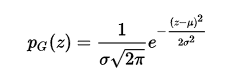2.椒盐噪声：椒盐噪声（salt-and-pepper
noise)又称脉冲噪声，它随机改变一些像素值，在二值图像上表现为使一些像素点变白，一些像素点变黑（可以理解为随机概率的像素点变色）。
3.泊松噪声：泊松噪声，就是符合泊松分布的噪声模型，泊松分布适合于描述单位时间内随机事件发生的次数的概率分布。
泊松分布公式（高中知识不解释了）：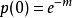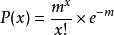几种噪声的对比：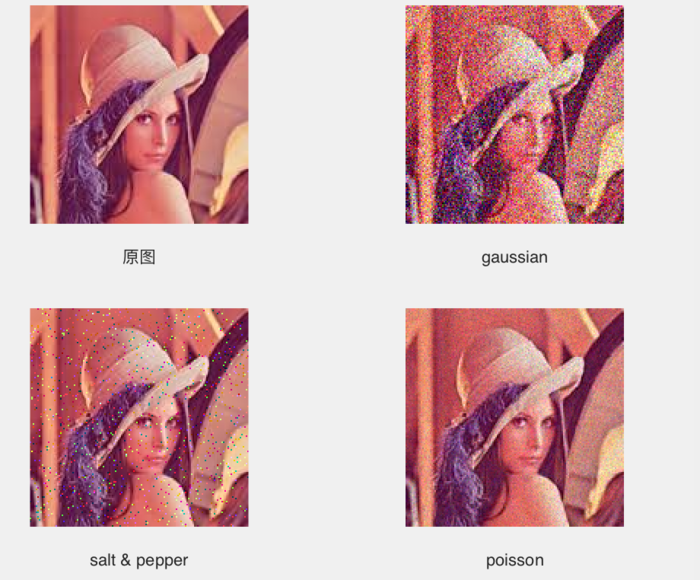(2)滤波：图像滤波，即在尽量保留图像细节特征的条件下对目标图像的噪声进行抑制，是图像预处理中不可缺少的操作，其处理效果的好坏将直接影响到后续图像处理和分析的有效性和可靠性。
针对不同噪声，也有不同的滤波方式：
1.高斯滤波-------顾名思义解决高斯噪声问题。
高斯滤波是一种线性平滑滤波，适用于消除高斯噪声，广泛应用于图像处理的减噪过程。通俗的讲，高斯滤波就是对整幅图像进行加权平均的过程，每一个像素点的值，都由其本身和邻域内的其他像素值经过加权平均后得到。方法主要有两种：
一是用离散化窗口滑窗卷积：就是选定一定大小的滑窗，遍历整个图像。比如我取像素9x9的矩阵滑窗，遍历整个图像进行卷积，一般我们都这样处理高斯噪声。
二是通过傅立叶变换。
只有当离散化的窗口非常大，用滑窗计算量非常大（即使用可分离滤波器的实现）的情况下，可能会考虑基于傅里叶变化的实现方法。
2.中值滤波-------解决椒盐噪声效果比较好。
中值滤波法是一种非线性平滑技术，它将每一像素点的灰度值设置为该点某邻域窗口内的所有像素点灰度值的中值。中值滤波的基本原理是把数字图像或数字序列中一点的值用该点的一个邻域中各点值的中值代替，让周围的像素值接近的真实值，从而消除孤立的噪声点。一般用于处理椒盐噪声。
3.均值滤波-------解决泊松噪声效果比较好。
均值滤波是典型的线性滤波算法，它是指在图像上对目标像素给一个模板，该模板包括了其周围的临近像素（以目标像素为中心的周围8个像素，构成一个滤波模板，即去掉目标像素本身），再用模板中的全体像素的平均值来代替原来像素值。

回答题目的问题：平滑处理就是用滤波器去除噪声！
2、使用matlab添加噪声
先做一个guide：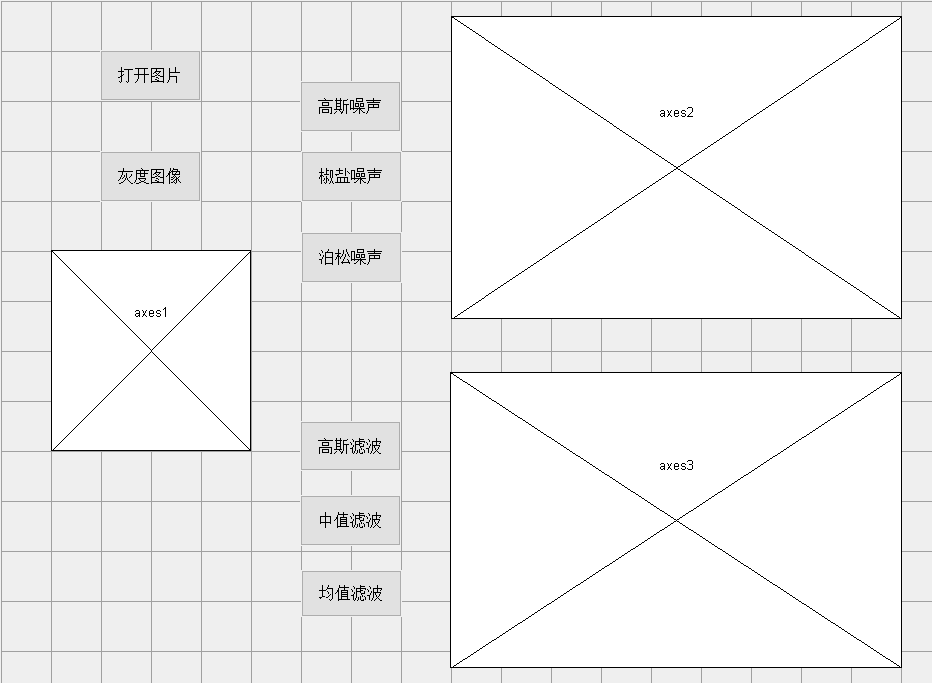打开图片和灰度代码：
% --- Executes on button press in 打开位图.
function openFile_Callback(hObject, eventdata, handles)
[filename,pathname]=uigetfile('*.bmp','select image');
str=[pathname filename];
guidata(hObject,handles);
axes(handles.axes1);
imshow(handles.I,handles.map);% --- Executes on button press in 转化灰度.
function togray_Callback(hObject, eventdata, handles)
handles.I=rgb2gray(handles.I);% 转灰度图像
guidata(hObject,handles);
axes(handles.axes1);
imshow(handles.I,handles.map);
原图：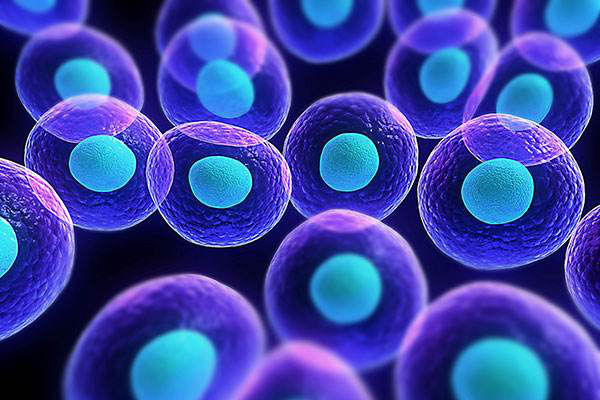（1）添加高斯噪声
% --- Executes on button press in 高斯噪声.
function guassian_Callback(hObject, eventdata, handles)
handles=guidata(hObject);
I2=handles.I;
handles.J=imnoise(I2, 'gaussian', 0,0.05);% 添加高斯噪声，方差值为0.01
guidata(hObject,handles);
axes(handles.axes2);
imshow(handles.J);效果：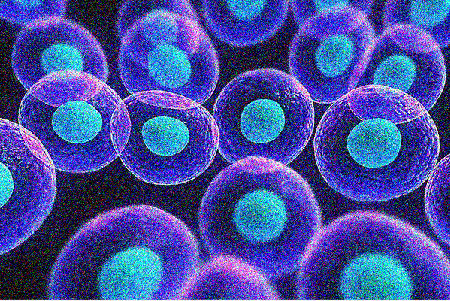（2）添加椒盐噪声：
% --- Executes on button press in  椒盐噪声.
function slat_Callback(hObject, eventdata, handles)
handles=guidata(hObject);
I2=handles.I;
handles.J=imnoise(I2, 'salt & pepper', 0.01);
guidata(hObject,handles);
axes(handles.axes2);
imshow(handles.J);效果：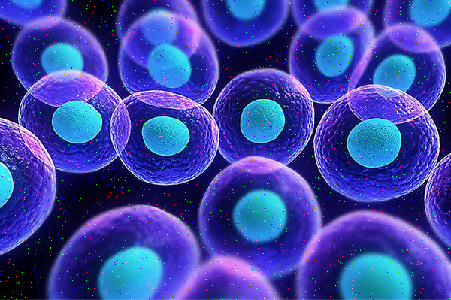（3）泊松噪声

% --- Executes on button press in 泊松噪声.
function poission_Callback(hObject, eventdata, handles)
handles=guidata(hObject);
I2=handles.I;
handles.J=imnoise(I2, 'poisson');
guidata(hObject,handles);
axes(handles.axes2);
imshow(handles.J);
效果：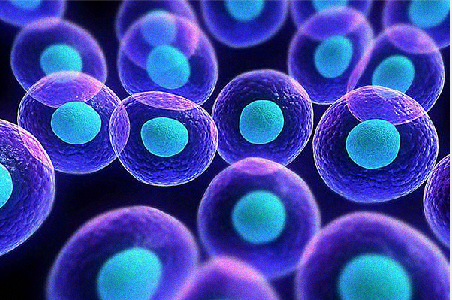3、平滑处理（图像滤波）

（1）对添加了高斯噪声的图像进行高斯滤波：
% --- Executes on button press in 高斯滤波.
function gaussianwave_Callback(hObject, eventdata, handles)
handles=guidata(hObject);
J2=handles.J;
grayImg=J2;
gausFilter = fspecial('gaussian',[5,5],1.6);
H=imfilter(grayImg,gausFilter,'replicate');
axes(handles.axes3);
imshow(H,handles.map);效果：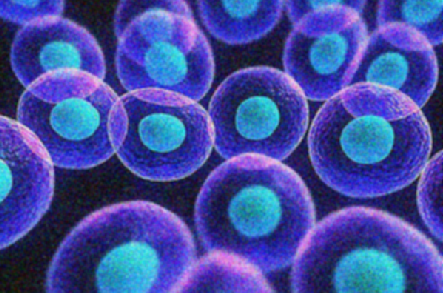（2）使用中值滤波处理椒盐噪声
% --- Executes on button press in 中值滤波.
function midwave_Callback(hObject, eventdata, handles)
handles=guidata(hObject);
J2=handles.J;
Z=medfilt2(J2,[3,3]);
axes(handles.axes3);
imshow(Z,handles.map);效果：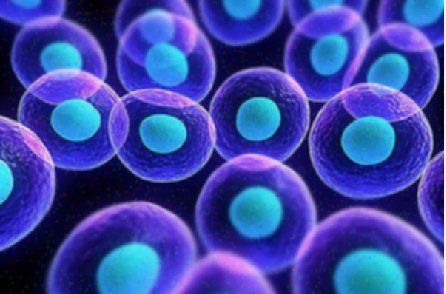（3）使用均值滤波处理泊松噪声
% --- Executes on button press in 均值滤波.
function avgwave_Callback(hObject, eventdata, handles)
handles=guidata(hObject);
J2=handles.J;
PSF=fspecial('average', 5);
P=imfilter(J2, PSF);
axes(handles.axes3);
imshow(P,handles.map);效果：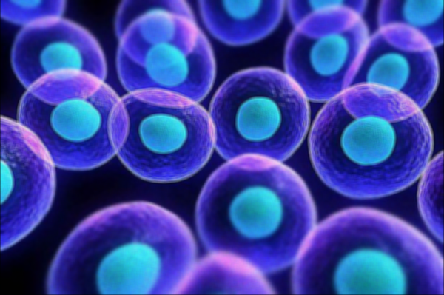展开全文matlab 滤波 图像处理
• 第一章概述图像概念：图：是物体透射或反射光的分布，客观存在 像：是人的视觉系统对图的接受在大脑中形成的印象或反映。 图像：是图和像的有机结合，是客观世界能量或状态以可视化形式在二维...数字图像处理的特点
第一章概述

图像概念：

图：是物体透射或反射光的分布，客观存在
像：是人的视觉系统对图的接受在大脑中形成的印象或反映。
图像：是图和像的有机结合，是客观世界能量或状态以可视化形式在二维平面上的投影。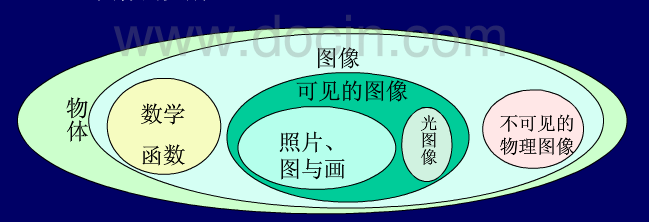图像处理的目的：

1）提高图像的视觉质量，达到赏心悦目的效果。
2）提高图像中所包含的某些特征或特殊信息，便于计算机分析
3）对图像数据进行交换、编码和压缩，便于图像的存储和传输。

数字图像处理的特点：

1）处理的数据量很大
2）处理占用的频带较宽。
3）各个像素的相关性大
4）不仅能完成线性计算，还能实现非线性计算，具有很高的灵活性。
数字图像处理与模拟图像处理相比的优点：

数字图像比模拟图像的优点：

数字图像不会因图像的存储、传输或复制等一系列操作二导致图像质量的退化，具有很好的再现性，主要优点是：精度高，再现性好，通用性、灵活性强。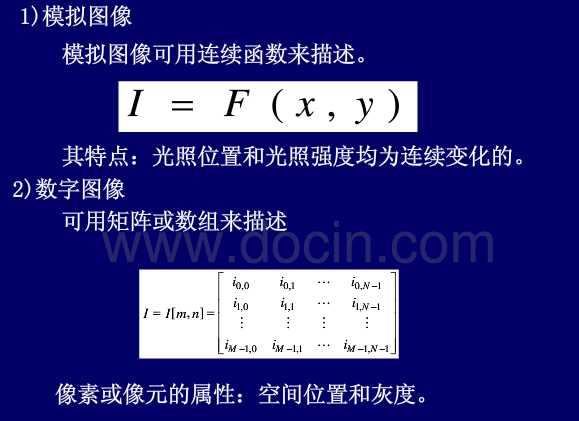数字图像处理系统结构：

图像数字化设备：扫描仪、数码相机、摄像机、图像采集器。
图像处理计算机：核心：图像处理计算机；附属：外储存器（硬盘、移动硬盘、光盘、闪盘的核心）
图像输出设备：打印机、显示器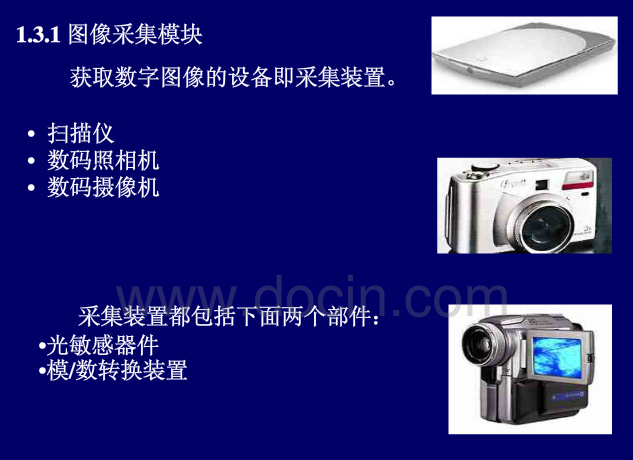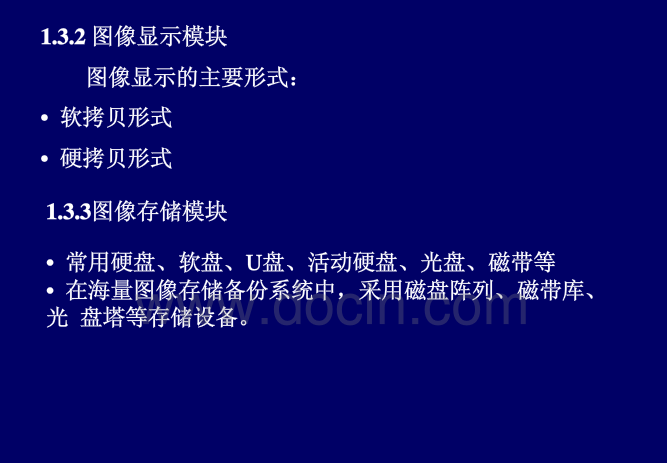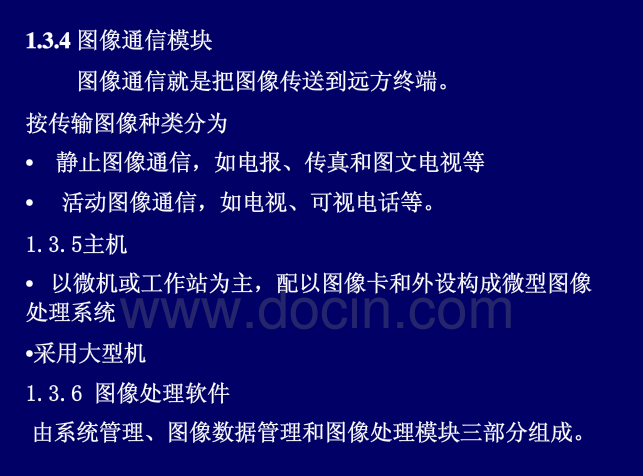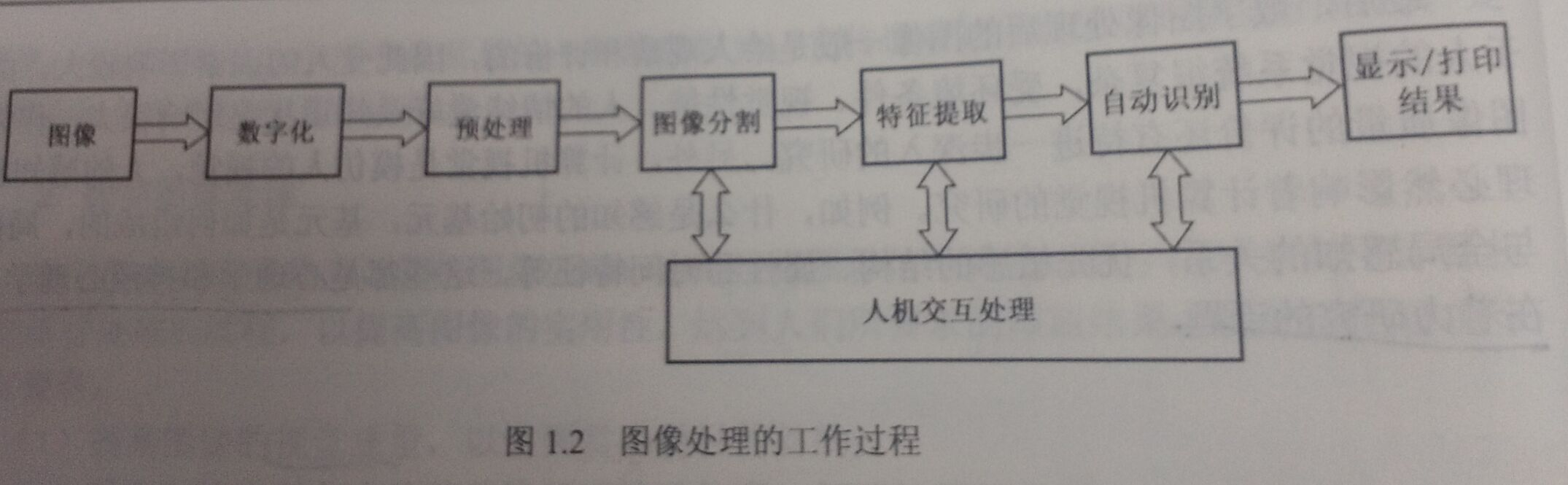具体描述：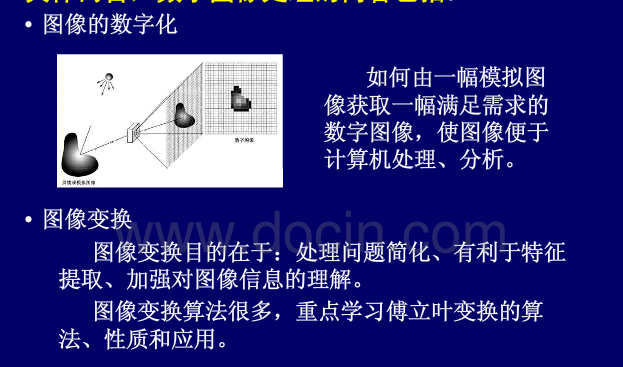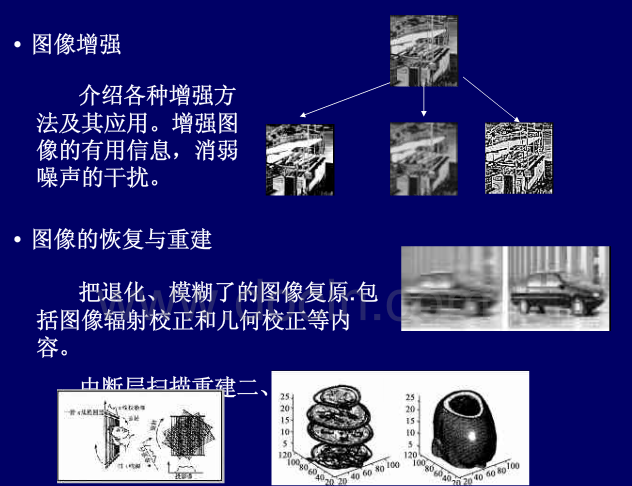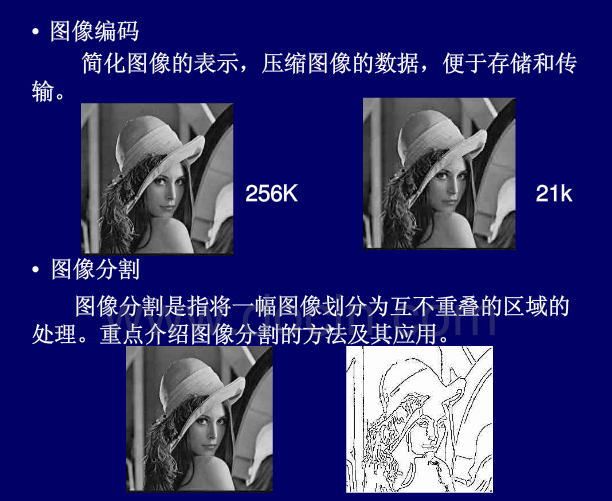数字图像处理主要研究的内容

1）图像增强：突出图像中人所感兴趣的部分
2）图像编码：1.减少数据存储量；2.降低数据率以减少传输宽带；3.压缩信息量，便于特征提取，为后续识别做好准备
3）图像复原：回复图像原貌
4）图像分割：按图像灰度或集合特性分割成若干区域的过程
5）图像分类：在图像经过预处理和特征分割、特征提取后，把不同类别的目标区分开来的图像处理方法。
6）图像重建：从数据到图像的处理过程。（例如CT）

数字图像处理的应用和发展

应用方面

1）航天和航空技术方面（图像复原、特征提取等）
2）生物医学工程方面（CT等）
3）通信工程方面（编码压缩）
4）工业和工程方面（图像识别）
5）军事、公安方面（图像分析、图像复原、图像识别等）
6）文化艺术方面
7）其他

发展

1）图像处理向着高速率、高分辨率、立体化、多媒体化、智能化、标准化发展
2）三维成像和多维成像
3）固化芯片更广阔的应用
4）算法的丰富和深入

第二章 数字图像处理基础

眼视觉系统

人的视觉过程：

1）光学过程：当人眼接收到光刺激时，首先是条件反射
2）化学过程：由视网膜神经进行处理，随后图像信号通过视觉通道反映到大脑皮层
3）神经处理过程：大脑皮层做出相应的处理;存储图像,信息处理、特征提取、决策和描述，最终做出反应。

马赫带效应：

是人类的视觉系统造成的，生物学对马赫带的解释是：人类的视觉系统有增强边缘对比度的机制。

图像的基本知识

1.图像的获取：

图像数字化：
将一幅圆面转化成计算机能处理的形式—数字图像的过程。具体说来，就是把一幅图画分割成一个个小区域(像元或像素)，并将各小区域灰度用整数来表示，形成一幅点阵式的数字图像。它包括采样和量化两个过程。像素的位置和灰度就是像素的属性。

1）采样：
将空间上连续的图像变换成离散点的操作称为采样。采砂间隔和采样孔径的大小是内个很重要的参数。采样方式:有缝、无缝和重迭

2）量化
经采样图像被分割成空间上离散的像素，但其灰度是连续的，还不能用计算机进行处理。将像素灰度转换成离散的整数值的过程叫量化。

采样量化原则： 1）对于缓变，应细量化，粗采样，以避免假轮廓 2）对于细节丰富的图像，应细采样，粗量化，以避免模糊（混叠）

量化等级越多，所得图像层次越丰富，灰度分辨率高，图像质量好，但数据量大;量化等级越少，图像层次欠丰富，灰度分辨率低，会出现假轮廓现象，图像质量变差，但数据量小。
但在极少数情况下对固定图像大小时，减少灰度级能改善质量，产生这种情况的最可能原因是减少灰度级一般会增加图像的对比度。例如 ：对细节比较丰富的图像数字化。

2.像素间的基本关系

1.邻接关系

N4（p）:（x+1,y）,（x-1,y）,（x,y-1）,（x,y+1）;
ND（p）:（x+1,y+1）,（x-1,y-1）,（x-1,y+1）,（x+1,y-1）.
4邻域 N4（p）
8邻域 N8（p） : N4（p）+ND（p）
4邻接：q在N4（p）中，具有v的两个像素 p, q 是4邻接。
8邻接：q在N8（p）中，具有v的两个像素 p, q 是8邻接。
m邻接:  1) q在N4（p）中，或者2) q在ND（p）中且集合N4（p）和N4（q）交集没有v值的像素，则具有v的两个像素 p, q 是m邻接.

1）4邻接必8邻接，反之不然
2）m邻接必8邻接，反之不然
3）m邻接是8邻接的变型，介于4邻接和8邻接之间，以消除8邻接中产生的歧义性。
*像素间同时存在4邻接和8邻接是优先4邻接。
2.距离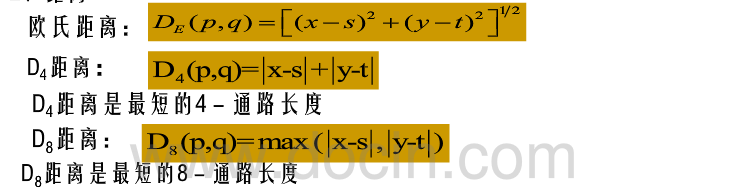图像分类

1）二值图像：图像的每个像素只能是黑或白，没有巾间的过渡，二值图像的像素值为0或1。
2）亮度图像：像素灰度值用8Bit表示，介于黑白之间的256种灰度中的一种。
3）索引图：颜色是预先定义好的，有256种颜色通过索引来表示，每个像素占8bit
4）RGB图像：真彩色图像，每个像素由红蓝绿三个字节组合而成，可产生1670万种不同颜色。

10.什么是图像灰度直方图? 图像直方图反映了图像的什么特征?
灰度的直方图反映图像中灰度级与出现这种灰度的概率之间关系的图形。这种图形能够反映图像灰度级的分布统计特征。

第三章图像基本运算

1.点运算

1）线性点运算

S=ar+b<!--//--><![CDATA[//><!--
S=ar+b
//--><!]]>

a=1,b=0,不变
a=1,b#0,灰度值上移或下移，图像变亮或暗
a>1,对比度变大
a<1,对比度降低
a<0,暗区变亮，亮区变暗。

2）非线性点运算

一般式：

S=c∗log（1+r）<!--//--><![CDATA[//><!--
S=c* log （1+r）
//--><!]]>
c为常数，r>=0.

S=c∗ry<!--//--><![CDATA[//><!--
S=c*r^y
//--><!]]>

y>1,高灰度的对比度增强
y<1,低灰度的对比度增强
y=1，不改变图像

2.代数运算

平均值去噪： Di（x,y）=Si（x,y）+Ni（x,y）<!--//--><![CDATA[//><!--
D_i（x,y）=S_i（x,y）+N_i（x,y）
//--><!]]>
S为理想图，N为噪音。
信噪比：P（x,y）=S2（x,y）/E[N2（x,y）]<!--//--><![CDATA[//><!--
P（x,y）=S^2（x,y）/E[N^2（x,y）]
//--><!]]>
对图做平均为： 平均D（x,y）=1/M∗sum（S（x,y）+Ni（x,y））<!--//--><![CDATA[//><!--
平均D（x,y）=1/M*sum（S（x,y）+N_i（x,y））
//--><!]]>
对应信噪比：P（x,y）=S2（x,y）/E[1/M∗sum（Ni（x,y））]2<!--//--><![CDATA[//><!--
P（x,y）=S^2（x,y）/E{[1/M*sum（N_i（x,y））]^2}
//--><!]]>
使得信噪比增加M倍。

减（Subtration）：
检测变化及运动的物体
乘（Multiplication）：
改变图像的灰度值，实现灰度级变换。
除（Division）：
改变图像的灰度值（比值图像处理）
3.逻辑运算

AND,OR,NOT

4.几何运算

平移 x1=x0+（−）X;y1=y0+（−）Y<!--//--><![CDATA[//><!--
x_1=x_0+（-）X ;   y_1=y_0+（-）Y
//--><!]]>
x（i,j）=I（i-a,j-b）#左右平移
镜像
【M，N】=size（I）
x（i,j）=I（i,N-j+1）#左右水平镜像
x（i,j）=I（M-i+1,j）#上下水平镜像
旋转（imrotate）
1) J=（I，60，’bilinear’）
2) J=（I，60，’bilinear’,’crop’）#截去局部
缩放
插值方法
1）最近邻插值法：缩小（取原图的偶数行，或取奇数行）；
2）插值算法
灰度重采样：

最近邻法（nearest）：取最近点的灰度值为点的灰度值
双线性插值法（bilinear）：考虑周围4个邻点的灰度值对它的影响。
f（u0,v0）=[f（u+1,v）−f（u,v）]∗a+[f（u,v+1）−f（u,v）]∗b<!--//--><![CDATA[//><!--
f（u_0,v_0）=[f（u+1,v）-f（u,v）]*a+[f（u,v+1）-f（u,v）]*b
//--><!]]>+[f（u+1,v+1）+f（u,v）−f（u,v+1）−f（u+1,v）]∗a∗b+f（u,v）<!--//--><![CDATA[//><!--
+[f（u+1,v+1）+f（u,v）-f（u,v+1）-f（u+1,v）]*a*b+f（u,v）
//--><!]]>
- 三次内插法（bicubic）：考虑周围16个邻点的灰度值对它的影响。

第四章图像转换

1.图像变换的目的:

①图像处理问题简化;
②有利于图像特征提取;
③有助于从概念上增强对图像信息的理解。
2.图像变换的要求

图像变换通常是一种二维正交变换。一般要求:
①正交变换必须是可逆的;
②正变换和反变换的算法不能太复杂;
③正交变换的特点是在变换域中图像能量将集中分布在低频率成分上，边缘、线状信息反映在高频率成分上，有利于图像处理。因此正交变换广泛应用在图像增强、图像恢复、特征提取、图像压缩编码和形状分析等方而。

3.傅立叶变换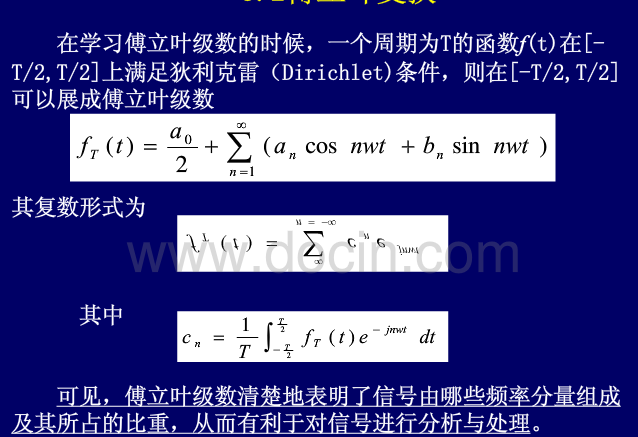3.1一维二维连续函数的傅立叶变换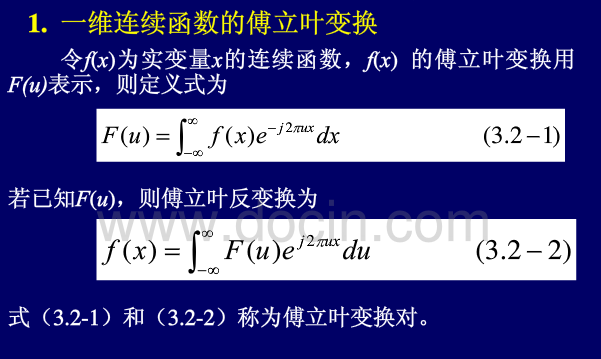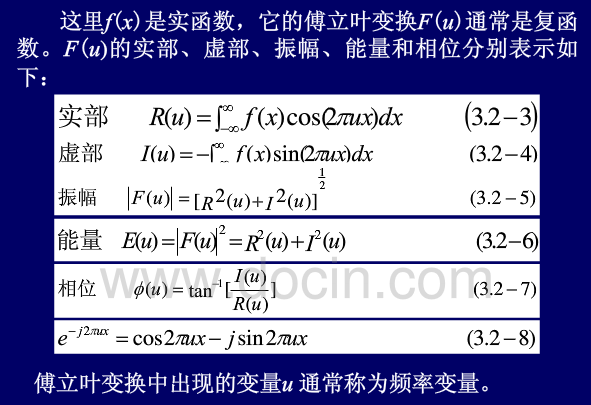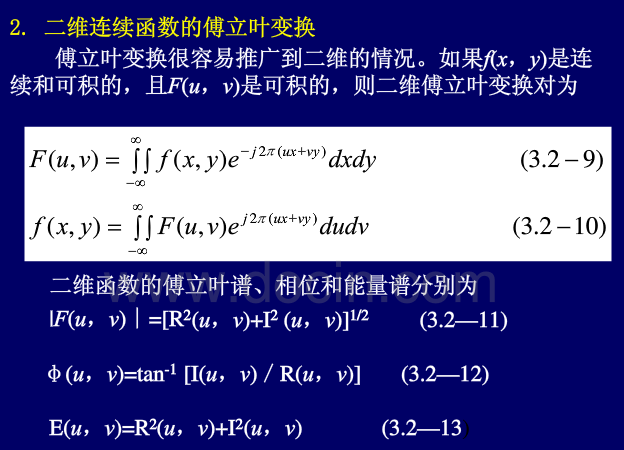3.2一维二维离散函数的傅立叶变换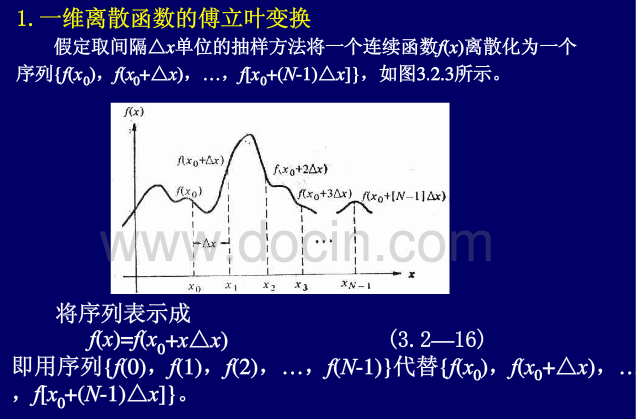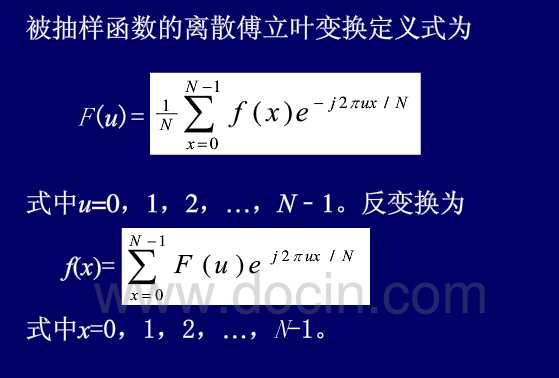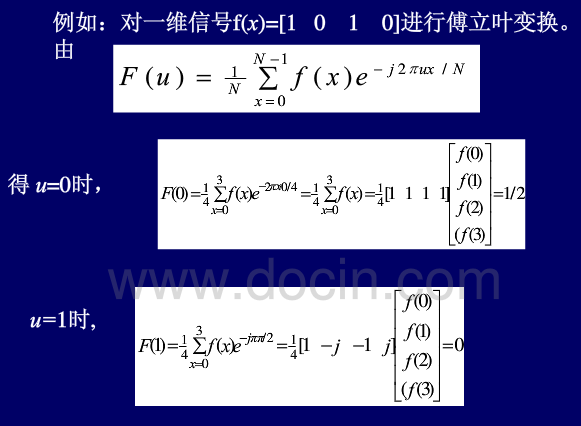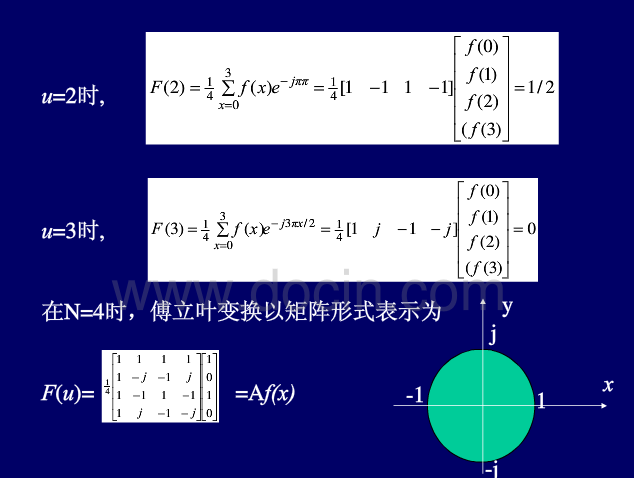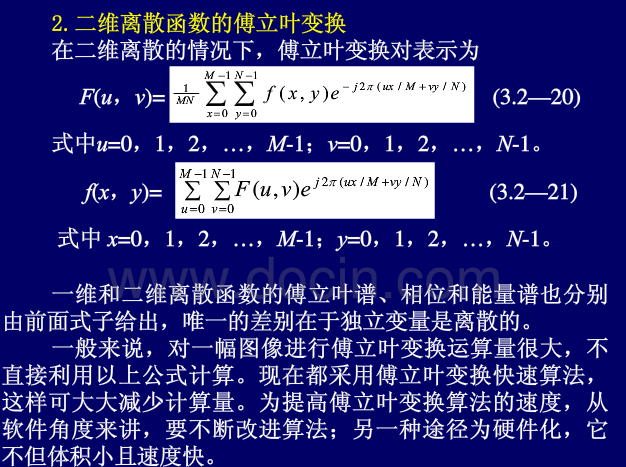4.二维离散傅立叶变换的若干性质

离散傅立叶变换建立了函数在空间域与频率域之间的转换关系。在数字图像处理中，经常要利用这种转换关系及其转换规律，因此，下而将介绍离散傅立叶变换的若下承要性质。

(1)周期性和共扼对称性
若离散的傅立叶变换和它的反变换周期为N，则有 F(u,v)=F(u+N，v)=F(u+，v+N)=F(u+N,v+N)<!--//--><![CDATA[//><!--
F(u,v)=F(u+N，v)=F(u+，v+N)=F(u+N,v+N)
//--><!]]>
傅立叶变换存在共扼对称性F(u,v)=F′(−u,−v)<!--//--><![CDATA[//><!--
F(u,v)=F'(-u,-v)
//--><!]]>这种周期性和共扼对称性对图像的频谱分析和显示带来很大益处。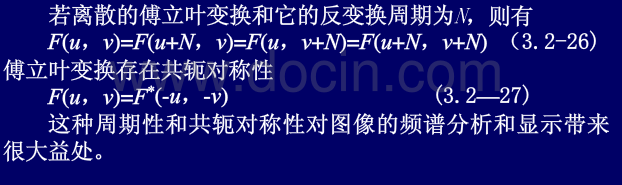(2)分离性
一个二维傅立叶变换可由连续两次一维傅立叶变换来实现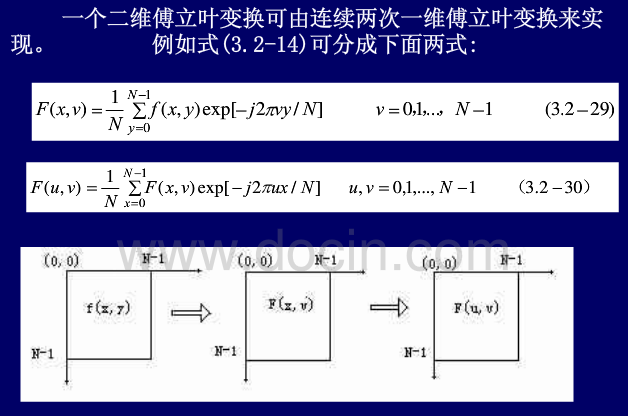(3)旋转性质
平面直角坐标写成极坐标形式.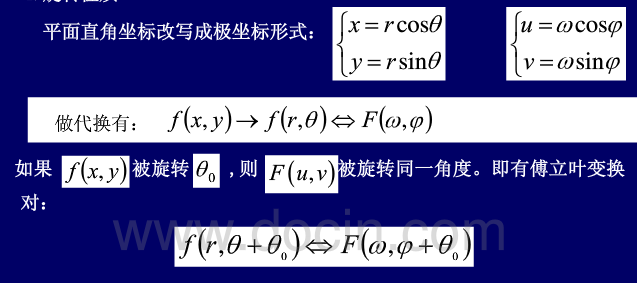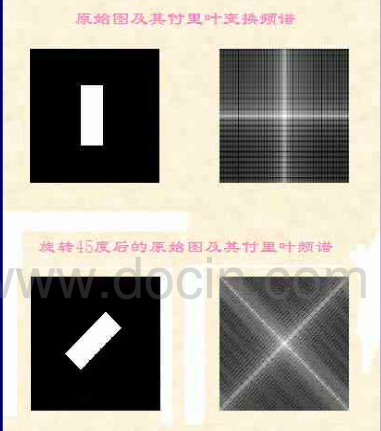5.卷积定理

f（u）∗g（u）=F（u）∗G（u）<!--//--><![CDATA[//><!--
f（u）*g（u）=F（u）*G（u）
//--><!]]>
展开全文图像变换 傅里叶变换 图像基本运算 matlab
• 本文中对于大多数的操作，是对数字图像处理领域中最为著名的“lena”图片进行操作的。原图如下（Figure 1）：  Figure 1 首先，在MATLAB中显示这幅图片： I=imread('lena.jpg'); imfinfo('lena.jpg') imsho.....
• 1.读入一副图像，对其进行缩放。 源代码： clear; % 读入图像函数imread(); % 参数：‘文件名/文件路径’ I=imread('lena.tif'); % 图像缩放函数imresize(); % 参数：图像对象，缩放倍率 I1=imresize(I,0.5...matlab
• 【高清原版】基于MATLAB数字图像处理技术应用数字图像处理
• b=0）、（a=1,b>0）和（a=1,b）等情况下的灰度变换处理，并比较处理前后的图像效果。 Matlab（matrixlab）矩阵实验室，在我的理解中matlab对灰度图片的提取即将其每个像素点的灰度提取，组成矩阵。 matlab代码： ...matlab 图像处理
• 在对图像进行锐化时，图像灰度变化之处将引入一段“过冲”，和人眼视觉中的马赫带效应相同。可以理解图像锐化即是增强了人眼的马赫带效应。 mat=zeros(300,500); mat(:,1:100)=0.2; mat(:,101:200)=0.3; mat(:,201...matlab 图像识别
• ## 数字图像处理——基于Matlab

万次阅读 多人点赞 2020-06-28 10:54:53
（注：本文代码大部分可从《数字图像处理 第三版》中找到）使用软件：MATLAB R2018a学习前提：了解matlab的GUI界面的每个按钮参考资料：《数字图像处理 第三版》，CSDN博客使用初音图片P站画师uid：1589657。...matlab 数字图像处理 GUI 学习笔记
• 写在前头：说到数字图像处理，不得不提起MATLAB。这是一款非常方便的仿真软件，绝大多数的图像处理可以用MATLAB完成。 　有人问，处理图片，用PS岂不是更好。两者各有优点，如果需要将1００００幅图片转换成灰度...图像处理 软件 matlab 图片
• 本书主要介绍MATLAB语言在图像处理技术方面的应用，重点介绍了各种图像处理方法的理论和实际应用，书中给出了大量MATLAB实例和图像处理效果，使渎职能够更好的理解和掌握使用MATLAB图像处理工具箱函数进行图像处理的...
• f = imread('F:\学习\研一上\数字图像处理\数字图像处理\作业1\rose.tif'); size (f) whos f imshow(f,[]) imwrite(f,'F:\学习\研一上\数字图像处理\数字图像处理\作业1\保存.tif') f1 = imread('F:\学习\研一上\...
• ## 基于matlab的数字图像处理GUI设计

万次阅读 多人点赞 2017-06-08 19:45:45matlab GUI 数字图像处理
• （一）matlab数字图像处理实验-guide的简单使用教程 （二）matlab数字图像处理实验-图像的几何变换 （三）matlab数字图像处理实验-图像灰度变换处理 （四）matlab数字图像处理实验-平滑处理 未完待续··...matlab 图像处理
• 1.6 MATLAB工作环境1.6.2使用编辑器创建m文件文本编辑器，也是图形MATLAB调试器，扩展符.m来表示。命令窗口输入edit打开文本编辑器。同样，输入edit filename会打开filename.m文件。如果不存在，会创图像处理 matlab matlab入门
• 本书利用MATLAB图像处理工具箱进行数字图像处理的设计应用，简洁明了地指出了所介绍的函数方法的理论背景，同时又紧密联系实际应用，以具体的实例说明了函数的使用方法。在实例中强调了如何用MATLAB图像处理工具...
• 赵小川《matlab数字图像处理实战》随书代码，及测试图片。matlab
• 数字图像处理图像增强MATLAB程序及仿真，带有源代码，数字图像处理必备。
• 为一幅图片添加椒盐噪声，高斯噪声，然后用均值滤波和中值滤波，进行滤波处理这两种噪声，比较两种滤波方式。 实验步骤 详见代码 实验结果 添加噪声 滤波结果 从实验结果看，对于椒盐噪声中值滤波...图像处理 Matlab
...